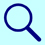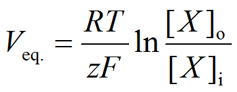PhysiologyWeb  Home  |  FAQ  |  About  |  Contact  |   - Sign InGlossary of Physiology TermsNernst equation
Definition:
An equation used to calculate the equilibrium potential (Veq.) of an ion. The equilibrium potential for an ion is also referred to as the Nernst potential for that ion. It is the membrane potential at which no net movement of the ion in question occurs across the membrane.where Veq. is the equilibrium potential, R is the universal gas constant, T is the temperature in Kelvin, z is the valence of the ionic species, F is the Faraday's constant, and [X]o and [X]i are the extracellular and intracellular, respectively, concentrations of the ion in question.